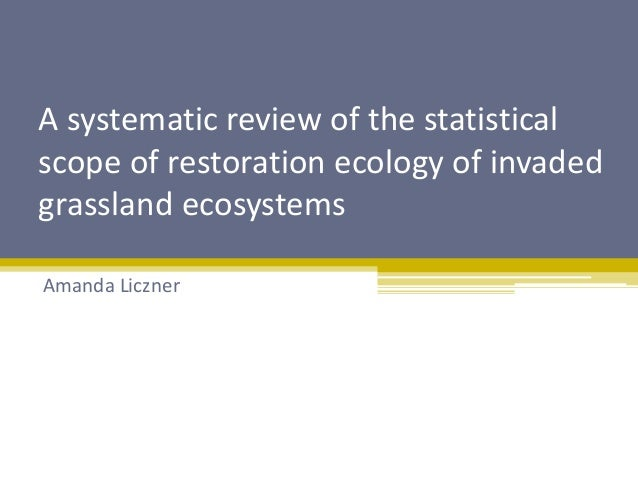Successfully reported this slideshow.Upcoming SlideShare
×

# Statistical scope of restoration ecology of invaded grasslands

586 views

Published on

2) The type of statistical tests used is dependent on the experimental design. A review by Mischener 1997 outlined common experimental designs used in restoration ecology and described appropriate statistical tests for each.
3) There has been a push to incorporate more variables in experimental designs to better explain systems. There is also movement away from ANOVAs towards Generalized Linear Models or GLMs. These movements have likely impacted the experimental designs, statistics and even the citation rate of restoration studies.
4) The purpose of this review is to look at the statistical scope of restoration ecology of invaded grasslands. I will examine the type of statistical tests being over time, whether assumptions are tested, and how statistical tests and the experimental design of studies influence the citation rate and the impact of the journal
5) A systematic literature search was conducted and resulted in 340 articles. Each article was individually screened to exclude reviews and irrelevant articles resulting in 103 retained articles
6) ANOVAs are the most commonly used statistical test comprising more than half of all tests. T-tests, non-parametric tests, and tests classified as ‘other’ are used less often.
7)ANOVAs have consistently been the most common statistical test. After 2000, the diversity of tests increased to include the diversity of tests observed today
8) The majority of studies tested the assumptions of the statistical tests used in their analyses.
9) Articles that performed more statistical tests have a citation rate and are published in higher impact journals. Increasing the number of factors has a negative impact on both the citation rate impact factor of journals.
10/11)The sample size of studies is positively correlated with both the average citation rate of the article and the impact of the journal where the article was published.
12) ANOVAs are likely the most common test as it is appropriate for many study designs used in restoration ecology. However, one assumption that ecological data infrequently meets without transformation is the assumption of normality. Thus, I recommend a shift towards GLMs which are appropriate for many study designs in restoration ecology and can handle non-normally distributed data
13) Although the majority of studies did test the assumptions of the statistical tests used, there is still many studies that did not test the assumptions which calls into question the interpretations of their results. Statistical models are also often poorly described in terms of how they defined, and this needs to be improved upon in future studies
14) Study design and statistics have an influence on the citation rate of articles and the impact factor of the journal where these articles are published. In order to increase the citation rate and journal impact, the sample size and number of tests should be increased. The number of factors should not be increased at the expense of sample

Published in: Science
• Full Name
Comment goes here.

Are you sure you want to Yes No• Be the first to comment

• Be the first to like this

### Statistical scope of restoration ecology of invaded grasslands

1. 1. A systematic review of the statistical scope of restoration ecology of invaded grassland ecosystems Amanda Liczner
2. 2. Introduction: experimental design dictates the type of statistics
3. 3. Introduction: the field of ecology is moving towards increased complexity
4. 4. Purpose: to describe the statistical scope of studies restoring invaded grasslands Most common statistical tests over time Statistical reporting Influence on citation rate and journal impact
5. 5. Methods: systematic literature search and PRISMA report Search terms: (restor*) AND (grass* OR savanna) AND (non-native* OR invas* OR invad* OR alien) Refined to “ecology”
6. 6. Results: The most common used test is the ANOVA
7. 7. Results: ANOVA remains the dominant stats test over time
8. 8. Results: the majority of studies tested the assumptions of the statistical tests used 60.9% tested assumptions
9. 9. Results: The number of stats tests, but not the number of factors, has a positive effect on citation rate and journal impact factor
10. 10. Results: citation rate is positively correlated with sample size
11. 11. Results: journal impact factor is positively correlated with sample size
12. 12. Discussion: a shift from ANOVAs to GLMs is recommended GLMs do not need a normally distributed response GLMs are appropriate for many study designs
13. 13. Discussion: statistical reporting needs improvement Assumptions must be tested Statistical models need to be appropriately described
14. 14. Discussion: statistics influences the citation rate and journal impact factor When possible sample size should be increased Number of factors should not be increased at the expense of sample size
15. 15. Conclusion: Shift from ANOVAs to GLMs To increase confidence we need to improve reporting Statistics and experimental design should be carefully chosen